Vibroengineering PROCEDIA

Published: 15 March 2019

# Modal analysis of micro wind turbine blade using COSMOSWorks

Xiaohua Tan1
Xiaosai Guo2
Xinbo Tan3
Lijie Wang4
Qiu Li5
Baizhou Li6
1, 2, 4, 5, 6Tianjin Key Lab of High Speed Cutting and Precision Machining, Tianjin University of Technology and Education, Tianjin, China
3Xiangtan Electric Power Co., Ltd., Xiangtan, Hunan, China
Corresponding Author:
Xinbo Tan
Views 113

#### Abstract

In this paper, vibration modal analysis of a horizontal axis micro wind turbine blade of different rotational speeds was carried out by using the finite element analysis software COSMOSWorks. The dynamic stiffening phenomenon and its effect on the vibration mode of the wind turbine blade were taken into account. Numerical results were analyzed and compared. The analysis can help not only to ensure the reliability of system operation but also to improve the structural performance.

## 1. Introduction

As an inexhaustible and renewable energy source, wind energy has been rapidly exploited in recent years. The wind turbine generates energy from the wind through its rotating blades. As one of the key components, the blade generally determines the performance and life of the wind turbine . The structure and strength of the blade plays an important role in the reliability of the wind turbine. Analysis of the dynamic characteristics of the wind turbine structure is an important aspect in the structural analysis of the wind turbine. Structural dynamics analysis of the construction machinery is mainly focused on the natural frequency and vibration mode characteristics . Structural dynamics analysis of wind turbine blades is more complicated than other common construction machineries . In recent years, the numerical method of modal analysis is widely used in wind turbine blade structural dynamics .

The blade structure is flexible due to its mechanical properties; therefore, vibration is inevitable when loaded by the randomly alternating wind. The blade undergoes tensile stress due to centrifugal force when spinning around its axis at high speed, which leads to dynamic stiffening phenomenon .

Micro wind turbines are usually referring to the ones that the rated power is less than 1 kW and the rated voltage range is between 12 V and 48 V . They have low requirements for wind energy resources and owning the advantages of compact structure and convenient installation. So far, there is still a lack of simulation and detailed comparison about the modal of micro wind turbine blades using COSMOSWorks. In this paper, a three-blade micro-wind turbine with 500 W rated power was studied. Modal analysis of the wind turbine blade was carried out by using the FEM software COSMOSWorks. The anisotropic mechanical properties of the FRP laminates and the dynamic stiffening effect of the blade were comprehensively considered, and the vibration modal simulation of the blade was calculated and analyzed.

## 2. Analytical theory of the blade modal

Each structure has an inherent vibration frequency, called resonant frequency. Once resonance frequency is activated, the structure will show a vibration statue, namely vibration mode. In structural static analysis, the node displacement is a main unknown quantity . The vibration equation is:

1
$\left[K\right]d=F,$

where $\left[K\right]$ is the stiffness matrix, $d$ is the unknown amount of node displacement, and $F$ is the known amount of node load. In dynamics analysis, damping matrix $\left[C\right]$ and mass matrix $\left[M\right]$ must be considered . Therefore, a typical damped oscillatory vibration equation is:

2
$\left[M\right]\stackrel{¨}{d}+\left[C\right]\stackrel{˙}{d}+\left[K\right]d=F\left(t\right),$

where $\stackrel{¨}{d}$, $\stackrel{˙}{d}$, $d$ and $F$ represent the finite element node acceleration, velocity, displacement and the role of external force vector respectively. When there is a lack of damping and external forces, the blades are free to vibrate at this time. Eq. (2) simplifies to:

3
$\left[M\right]\stackrel{¨}{d}+\left[K\right]d=0.$

Since there is no external load, the solution of the equation reflects the inherent properties of the structure, namely frequency and vibration mode [9, 10]. Eq. (3) can be converted to:

4
$\left[K\right]\left\{\mathrm{\Phi }\right\}=\lambda \left[M\right]\left\{\mathrm{\Phi }\right\}.$

Eq. (4) is the generalized eigenvalue equation of the blade structural dynamics. The unknowns in the formula are the eigenvalues and eigenvectors of the leaf structure .

Due to the coupling of large displacement and small deformation, flexible mechanical system usually exhibits dynamic stiffening phenomenon . Miniature wind turbine blade is an elastic mechanism manufactured by FRP composite material, it will subject to centrifugal force when the impeller rotates around the shaft at high speed. The tensile stress caused by centrifugal force will affect the blade deformation. That is, the interaction between the rotation of the shaft and the deformation of the blade leads to the increase in the blade rigidity, which is the so-called Dynamic Stiffening.

## 3. Finite element model

Wind turbine blades are commonly manufactured by special composite material composed of two or more different materials with different properties. Its main advantage is high specific stiffness (ratio of height and weight). Due to orthotropic, it is necessary to define the material properties in different directions. Commonly used in wind turbine blades, Fiber Reinforced Plastic Material (FRPM) owns the advantages of light weight, corrosion resistance and designable rigidity. The mechanical properties of the FRP composite material also differ with the compound ratio. The material properties studied in this paper are as shown in Table 1.

Table 1Material properties of the blade

 Density $\rho$ Poisson’s ratio $\mu$ Elastic modulus $E$ (Pa) Shear elastic modulus $G$ (Pa) Spanwise Radial Spanwise Radial 1.95×103 0.22 4.26×1010 2.76×1010 1.5×1010 1.5×1010

In this paper, a suitable airfoil shape was selected by Profili software, and the airfoil data and aerodynamic parameters was obtained in the meantime. The blade was divided into several sections. Basing on Glauert theory of blade design, the chord length, mounting angle and airflow angle were calculated by using Matlab programming. Then the actual three-dimensional coordinates of each section of the blade were obtained basing on the point coordinate transformation. Finally, SolidWorks software was applied for solid modeling .

Fig. 1The three-dimensional model of wind turbine blade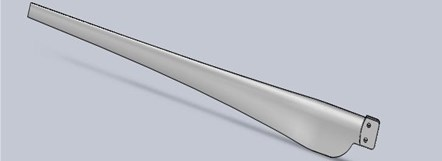As a build-in finite element analysis software of SolidWorks, COSMOSWorks can seamlessly import model without losing blade features. Therefore, the blade modal analysis was carried out under the SolidWorks interface environment. During the mesh procedure of finite element analysis, tetrahedral elements were used for the solid geometry partition, which can guarantee a credible meshing. There are four nodes for each tetrahedron, corresponding to the four corners of the tetrahedron. Each node has 3 degrees of freedom, which means that the node displacement can be completely represented by 3 components.

Fig. 2The mesh diagram used for the FEM model of the blade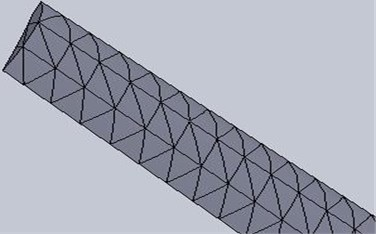The natural frequency and vibration mode of components mainly depend on their stiffness distribution and mass distribution. Therefore, the modal analysis of the blade only needs to define the constraints. Two types of constraints are commonly used for blade. The first is the complete restraint of the entire root cross-section. The other is to impose constraints on the width of the support section in the root section, i.e. without considering the transition surface, only the extent of the support section is considered to be a constraint on the blade. Taking into account that this study involves different speeds under the influence of dynamic stiffening on the blade, the second method was adopted. The constraint on the petiole part is shown in Fig. 3.

Fig. 3The fixed constraint on the blade handle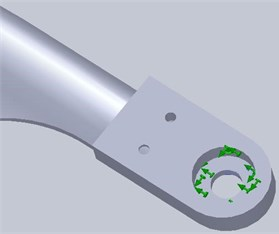## 4. Result calculation and discussion

In order to analyze the influence of centrifugal force on the stiffness, the first ten modes of modal analysis and the modal comparison of the blades at different rotational speeds were carried out. The obtained modal of a statical blade and a blade rotating at its rated speed are shown in Fig. 4 and Fig. 5 respectively.

Fig. 4Modal analysis diagram of a statical blade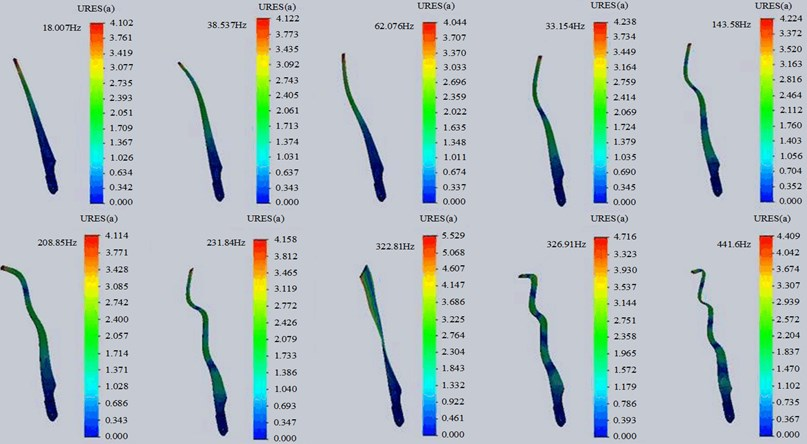Fig. 5Modal analysis diagram of a blade rotating at the rated speed of 360 rpm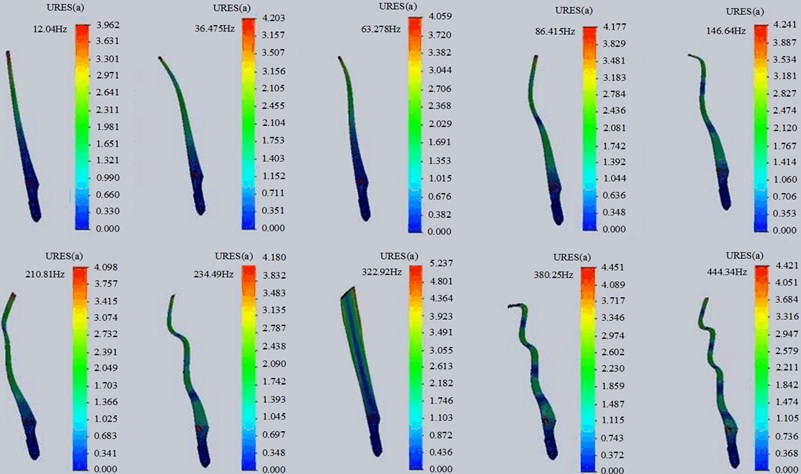It can be seen from the results that vibration mode from the first to the seventh order was mainly composed by waving and bending vibration. While the high-order vibration mode is a combination of bending and torsion. That is, the main vibration mode of the blade is bending mode. According to vibration theory, the energy during the vibration process is mainly concentrated on the first two order, which mainly showed waving and bending vibration in this case. It can be seen that the waving vibration has a great influence on the blade, and the influence of torsional vibration is relatively small. A detailed comparison of modal analysis results of blades at different speeds is listed in Table 2.

In this study, the rated speed of the blade is 360 rpm, which is about 6 Hz. While the minimum resonant frequency of a stationary blade is 10.007 Hz. The natural frequency of rotating blade is different from the static blade. This is because the blade is subjected to tensile stress due to centrifugal force, which increases blade rigidity. The increased stiffness affects the blade's resonant frequency, and makes the blade rotate more stable.

Table 2Comparison of modal analysis results of blades at different speeds

 Frequency(Hz) RPM 1 100 200 360 450 550 650 Group 1 10.006 10.259 10.977 12.84 14.138 15.706 17.356 Group 2 33.534 33.772 34.474 36.475 38.006 39.981 42.184 Group 3 62.083 62.178 62.462 63.278 63.913 64.752 65.722 Group 4 83.74 83.948 84.568 86.415 87.906 89.93 92.322 Group 5 143.57 143.81 144.53 146.64 148.34 150.62 153.31 Group 6 208.9 208.99 209.25 210.01 210.6 211.37 212.23 Group 7 231.83 232.04 232.65 234.49 236 238.09 240.61 Group 8 322.58 322.61 322.69 322.92 323.1 323.34 323.63 Group 9 327.09 327.33 328.06 330.25 332.03 334.45 337.33 Group 10 441.63 441.88 442.63 444.84 446.63 449.06 451.95

## 5. Conclusions

A modal analysis of a three-blade wind turbine blade was carried out by using COSMOSWorks in this paper. Through the modal analysis of blades, it can be concluded that the obtained minimum frequency of rotating blade is greater than the frequency obtained in static state due to the role of dynamic stiffening. Therefore, resonance can be effectively avoided, and ensures safe and reliable operation of the system.

#### Acknowledgements

This work was financially supported by the National Natural Science Foundation of China (No. 11702193 and No. 11772227), Scientific Research Project of Tianjin Municipal Education Commission (No. 2017KJ108 and No. 2017KJ113), the Research Project of Tianjin University of Technology and Education (No. KYQD1702 and No. KJ1705), and the Training Plan for Young and Middle-aged Backbone Talents of Tianjin Universities and Colleges (No. RC180204).